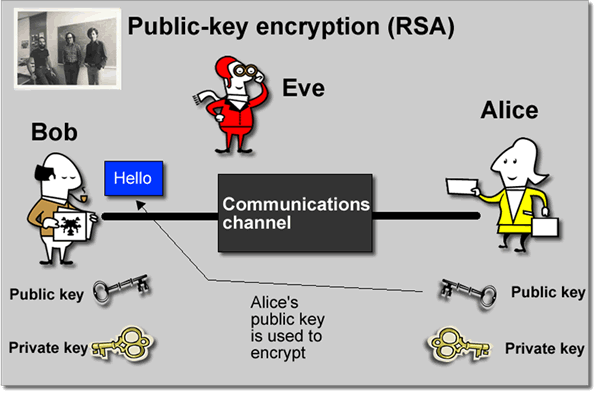## Sunday, June 29, 2014

### Why my encrypted value is different each time?A few days ago, one guy at work asked me when using a crypto wrapper that I have written, why the encrypted value is different each time? For that crypto wrapper, I was simply using the C# RSACryptoServiceProvider , with a cert file as public key and a password protected pfx file containing the private key.

Well, it uses RSA  algorithm, and I think my colleague must have learned this at school, long time ago ;). The standard text-book RSA algorithm does not use padding so the encrypted value gets generated is same each time. That pretty much makes RSA not secure in real world because hackers can try different combinations to reproduce the same value instead of brute force solving what are the two prime numbers.

With that said, let’s review the basic of RSA in case you get asked some related questions in your interview. I am not a security expert in any means, just the most basic stuff.

1) Find two prime number, prefer large one, more than 100 bits, for example, P and Q, and let
N = P * Q
M = (P - 1) * (Q - 1)
2) Find a number E that is a relative prime of M, meaning the Greatest Common Divisor (gcd) of E and M is 1.
3) Find a number D, so that E * D mod M = 1.

With the above, now you have a public key E to encrypt your data, and you have your private key D to decrypt. N is also public.

For example, now you want to encrypt data X,
X^E mod N = Y

According to Fermat’s little theorem, you could decrypt Y with private D using
Y^D mod N = X

It is proved that without D, it will take extremely long time to figure out the two prime numbers given N. Until this point, if you are using the above method, the encrypted value should be the same every time using public key E. This is where padding coming into picture.

Take OAEP (Optimal Asymmetric encryption padding) Padding , it adds different numbers to the X before encrypting it so that the encrypted values are different each time. In decryption, it removes those values.

An interesting article written (in Chinese) by Dr. Jun Wu, while he was still at Google. If you can read Chinese, highly recommend you to read it.
http://www.kuqin.com/math/20071204/2796.html . His original blog was shut down, this was saved by some other people, you can also take a look at his book “数学之美” 

Reference:
 RSA:
 OAEP
 数学之美